Courses

# NABARD Manager Reasoning Mock Test 5

## 20 Questions MCQ Test NABARD Manager - Mock Tests & Previous Year Papers | NABARD Manager Reasoning Mock Test 5

Description
This mock test of NABARD Manager Reasoning Mock Test 5 for Banking Exams helps you for every Banking Exams entrance exam. This contains 20 Multiple Choice Questions for Banking Exams NABARD Manager Reasoning Mock Test 5 (mcq) to study with solutions a complete question bank. The solved questions answers in this NABARD Manager Reasoning Mock Test 5 quiz give you a good mix of easy questions and tough questions. Banking Exams students definitely take this NABARD Manager Reasoning Mock Test 5 exercise for a better result in the exam. You can find other NABARD Manager Reasoning Mock Test 5 extra questions, long questions & short questions for Banking Exams on EduRev as well by searching above.
QUESTION: 1

### Which of the following expressions will be true if the expression 'H ≤ G = K > F is definitely true?

Solution: H ≤ G = K > F
(A) K ≤ H : (True)
(B) G = F : (Not True)
(C) H ≤ F : (Not True)
(D) H ≥ G : (Not True)
QUESTION: 2

Solution:
QUESTION: 3

### The senate candidate expressed outrage that few judges have any background in technology, yet they try to resolve cases involving high tech companies. He stated that not one federal judge has a degree or any experience in computer technology. A promising response to this concern, arguing that things are not as bad as they might seem, could involve which of the following claims?

Solution:
QUESTION: 4

Opponents of laws that require automobile drivers and passengers to wear seat belts argue that in a free society people have the right to take risks as long as the people do not harm others as a result of taking the risks. As a result, they conclude that it should be each person's decision whether or not to wear a seat belt.
Which of the following, if true, most seriously weakens the conclusion drawn above?

Solution:
QUESTION: 5

In the following questions, the symbols @, # \$, ✹ and % are used as illustrated below:
'P @ Q' means 'P is not smaller than Q'.
'P # Q' means 'P is neither grater than nor equal to Q'.
'P \$ Q' means 'P is neither smaller than nor greater than Q'.
'P ✹ Q' means 'P is not greater than Q'.
'P % Q' means 'P is neither smaller than nor equal to Q'.
Now in each of the following questions assuming the given statements to be true, find which of the two conclusions I and II given below them is/are definitely true.

Statement:
M \$ K, D ✹ K, R # K
Conclusions:
I. D \$ M
II. M % D

Solution:

M = K ....(i); D≤ K ....(ii)
R < .....(iii)
From (i) and (ii), we get
M = K ≥ D ⇒⇒ M ≥ D
Hence, either M > D (conclusion II) or M = D (conclusion I) is true.

QUESTION: 6

In the following questions, the symbols @, # \$, ✹ and % are used as illustrated below:
'P @ Q' means 'P is not smaller than Q'.
'P # Q' means 'P is neither grater than nor equal to Q'.
'P \$ Q' means 'P is neither smaller than nor greater than Q'.
'P ✹ Q' means 'P is not greater than Q'.
'P % Q' means 'P is neither smaller than nor equal to Q'.
Now in each of the following questions assuming the given statements to be true, find which of the two conclusions I and II given below them is/are definitely true.

Statement:
F ✹ M, M % R, E @ F
Conclusions:
I. M % E
II. R @ E

Solution:

F ≤ M ...(i); M > R ...(ii); E ≥ F ... (iii)
From (i) and (iii), no specific relation can be obtained between M and E. Similarly, no specific relation can be obtained between R and E.

QUESTION: 7

A, B, C, D, E, F, G and H are sitting round the circle and are facing the centre:
(i) A is second to the right of E who is the neighbour of C and G.
(ii) D is not the neighbour of A ?
(iii) G is the neighbour of F
(iv) B is not between D and H. H is not between F and D.

Q. What is the position of D?

Solution: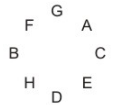QUESTION: 8

A, B, C, D, E, F, G and H are sitting round the circle and are facing the centre:
(i) A is second to the right of E who is the neighbour of C and G.
(ii) D is not the neighbour of A ?
(iii) G is the neighbour of F
(iv) B is not between D and H. H is not between F and D.

Q. Which two of the following are not neighbours?

Solution:QUESTION: 9

A, B, C, D, E, F, G and H are sitting round the circle and are facing the centre:
(i) A is second to the right of E who is the neighbour of C and G.
(ii) D is not the neighbour of A ?
(iii) G is the neighbour of F
(iv) B is not between D and H. H is not between F and D.

Q. Which of the following is correct?

Solution:QUESTION: 10

Each question below consists of two statements numbered I and II . You have to decide whether the data provided in the statements are sufficient to answer the questions.
Give answer (1) if the statement I alone is sufficient to answer the question, but the statement II alone is not sufficient.
Give answer (2) if the statement II alone is sufficient to answer the question, but the statement I alone is not sufficient.
Give answer (3) if the data either in statement I alone or in statement II alone are sufficient to answer the question.
Give answer (4) if you cannot get the answer from the statement I and II together, but need even more data.
Give answer (5) if the data in both statements I and II together are necessary to answer the question.

Q. What was the length of a running train crossing another 180 metre long train running in the opposite direction?
I. The relative speed of the two trains was 150 kmph.
II. The train took 9 seconds to cross each other.

Solution:

Let the two trains of length a metres and b metres be moving in opposite directions at u m/s and v m/s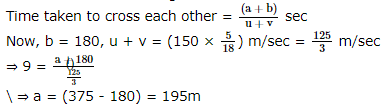QUESTION: 11

Each question below consists of two statements numbered I and II . You have to decide whether the data provided in the statements are sufficient to answer the questions.
Give answer (1) if the statement I alone is sufficient to answer the question, but the statement II alone is not sufficient.
Give answer (2) if the statement II alone is sufficient to answer the question, but the statement I alone is not sufficient.
Give answer (3) if the data either in statement I alone or in statement II alone are sufficient to answer the question.
Give answer (4) if you cannot get the answer from the statement I and II together, but need even more data.
Give answer (5) if the data in both statements I and II together are necessary to answer the question.

Q.By selling a product for Rs.100, how much profit was earned?
I. 20% profit would have been earned if it was sold for Rs.90.
II. The profit was one-third of the purchase price.

Solution: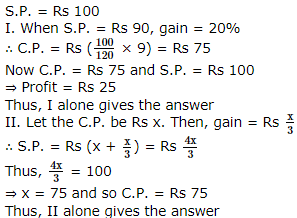QUESTION: 12

Each question below consists of two statements numbered I and II . You have to decide whether the data provided in the statements are sufficient to answer the questions.
Give answer (1) if the statement I alone is sufficient to answer the question, but the statement II alone is not sufficient.
Give answer (2) if the statement II alone is sufficient to answer the question, but the statement I alone is not sufficient.
Give answer (3) if the data either in statement I alone or in statement II alone are sufficient to answer the question.
Give answer (4) if you cannot get the answer from the statement I and II together, but need even more data.
Give answer (5) if the data in both statements I and II together are necessary to answer the question.

Q. What was the cost price of the suitcase purchased by Richard?
I. Richard got 20% concession on the labelled price.
II. Richard sold the suitcase for Rs.2000 with 25% profit on the labelled price.

Solution: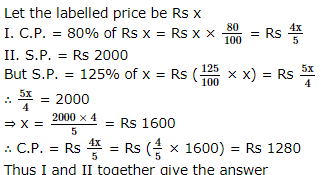QUESTION: 13

Five girls are sitting on a bench to be photographed. Seema is to the left of Rani and to the right of Bindu. Mary is to the right of Rani. Reeta is between Rani and Mary.

Q. Who is sitting immediate right to Reeta ?

Solution: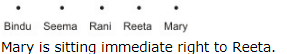QUESTION: 14

Five girls are sitting on a bench to be photographed. Seema is to the left of Rani and to the right of Bindu. Mary is to the right of Rani. Reeta is between Rani and Mary.

Q. Who is in the middle of the photograph ?

Solution: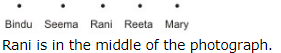QUESTION: 15

A word arrangement machine, when given a particular input, rearranges it following a particular input, rearranges it following a particular rule. The following is the illustration of the input and the steps of arrangement:
Input: cri end yam stu che
Step-I: yam che cri end stu
Step-II: yam che stu end cri
Step-III: stu cri yam che end
Step-IV: stu cri end che yam
And so on goes the machine. Study the logic and answer the questions that follow:

Q. If Step-VII of an Input is "over the pre new bone", what is Step-IV of that Input?

Solution:
QUESTION: 16

A word arrangement machine, when given a particular input, rearranges it following a particular input, rearranges it following a particular rule. The following is the illustration of the input and the steps of arrangement:
Input: cri end yam stu che
Step-I: yam che cri end stu
Step-II: yam che stu end cri
Step-III: stu cri yam che end
Step-IV: stu cri end che yam
And so on goes the machine. Study the logic and answer the questions that follow:

Q. Given the following Input: "sym reach lad photo cal", what Step will be the following arrangement?
Arrangement: "reach lad photo sym cal"

Solution:
QUESTION: 17

A word arrangement machine, when given a particular input, rearranges it following a particular input, rearranges it following a particular rule. The following is the illustration of the input and the steps of arrangement:
Input: cri end yam stu che
Step-I: yam che cri end stu
Step-II: yam che stu end cri
Step-III: stu cri yam che end
Step-IV: stu cri end che yam
And so on goes the machine. Study the logic and answer the questions that follow:

Q. If Step-VI of a given Input be "map ind pak rus eng", what would be the Input?

Solution:
QUESTION: 18

Below are the statements followed by four conclusions numbered I, II, III and IV. You have to consider the statements and the following conclusions and decide which of the conclusion(s) follows the statement(s).

Statements:
a. All rockets are poles.
b. Some poles are trams.
c. Some trams are ropes.
d. All ropes are tents,
Conclusions:
I. Some tents are trams.
II. Some ropes are rockets.
III. Some trams are rockets.
IV. Some poles are rockets.

Solution:
QUESTION: 19

Below are the statements followed by four conclusions numbered I, II, III and IV. You have to consider the statements and the following conclusions and decide which of the conclusion(s) follows the statement(s).

Statements:
a. All dials are mirrors.
b. All mirrors are spoons.
c. Some spoons are decks.
d. Some decks are chairs.
Conclusions:
I. Some decks are mirrors.
II. Some spoons are dials.
III. Some decks are dials.
IV. Some chairs are spoons.

Solution:
QUESTION: 20

Below are the statements followed by four conclusions numbered I, II, III and IV. You have to consider the statements and the following conclusions and decide which of the conclusion(s) follows the statement(s).

Statements:
a. Some houses are forests.
b. All forests are trees.
c. Some trees are hills.
d. All hills are buses.
Conclusions:
I. Some buses are trees.
II. Some trees are houses.
III. Some hills are houses.
IV. Some buses are forests.

Solution: